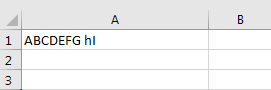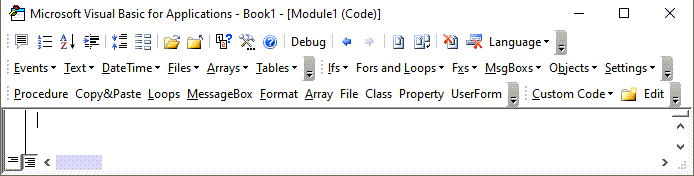# VBA Right Function – Extract Text From Right

This tutorial will demonstrate how to use the Right VBA function to extract text from the right.

## Right Function

### VBA Right function Last n Characters

The VBA Right function returns the last n characters from a string:

``````Sub RightExample_1()
MsgBox Right("ABCDEFGHI", 4)         'Result is: "FGHI"
MsgBox Right("ABCDEFGHI", 2)         'Result is: "HI"
MsgBox Right("ABCDEFGHI", 1)         'Result is: "I"
MsgBox Right("ABCDEFGHI", 100)       'Result is: "ABCDEFGHI"
End Sub``````

### VBA Right Function Last n Characters in a Variable

As shown above, you can define a string simply by entering text surrounded by quotation marks. But the RIGHT Function will also work with string variables. These examples will extract the last n characters from a string variable.

``````Sub RightExample_2()
Dim StrEx As String 'Define a string variable
StrEx = "ABCDEFGHI"

MsgBox Right(StrEx, 4)                'Result is: "FGHI"
MsgBox Right(StrEx, 2)                'Result is: "HI"
MsgBox Right(StrEx, 1)                'Result is: "I"
MsgBox Right(StrEx, 100)              'Result is: "ABCDEFGHI"
End Sub``````

### VBA Right Function Last n Characters from a Cell

Strings can be defined in VBA code but also you can use values from cells. Read the value of a cell, keep it in a string variable, and extract last n characters from that Worksheet Cell value.``````Sub RightExample_3()
Dim StrEx As String 'Define a string variable
'Read the value of cell A1 in worksheet Sheet1
StrEx = ThisWorkbook.Worksheets("Sheet1").Range("A1").Value

'For this example the value of cell A1 is "ABCDEFG hI"

MsgBox Right(StrEx, 4)   'Result is: "G hI"
MsgBox Right(StrEx, 2)   'Result is: "hI"
MsgBox Right(StrEx, 1)   'Result is: "I"
MsgBox Right(StrEx, 100) 'Result is: "ABCDEFG hI"
End Sub``````

### VBA Right Function Trim off First Letter

To remove letters from the beginning of a string, use the RIGHT Function along with the LEN Function.

The VBA LEN function counts the number of characters in a string:

``Len(StrEx)``

By combining the functions, we can remove a certain number of characters from the start of the string:

``````Sub RightExample_4()
Dim StrEx As String 'Define a string variable
StrEx = "ABCDEF"

MsgBox Right(StrEx, Len(StrEx))     'Result is: "ABCDEF"
MsgBox Right(StrEx, Len(StrEx) - 1) 'Result is: "BCDEF"
MsgBox Right(StrEx, Len(StrEx) - 2) 'Result is: "CDEF"
End Sub``````

### VBA Right to Extract Text after Space

To extract the last name from a string with a full name, use the Right Function along with Len and Instr Function.

The VBA LEN function counts the number of characters in a string:

``Len(StrEx)``

The VBA InStr function searches for a substring inside a string and returns the position number of the substring.

``InStr(StrEx, " ")``

By combining the functions, we can extract the part after the first space from a phrase:

``````Sub RightExample_5()
Dim StrEx As String 'Define a string variable
StrEx = "Luke Skywalker"

MsgBox Right(StrEx, Len(StrEx) - InStr(StrEx, " "))
'Result is: "Skywalker"

StrEx = "Leonardo da Vinci"
MsgBox Right(StrEx, Len(StrEx) - InStr(StrEx, " "))
'Result is: "da Vinci"

StrEx = "May the Force be with you"
MsgBox Right(StrEx, Len(StrEx) - InStr(StrEx, " "))
'Result is: "the Force be with you "
End Sub``````

Stop searching for VBA code online. Learn more about AutoMacro - A VBA Code Builder that allows beginners to code procedures from scratch with minimal coding knowledge and with many time-saving features for all users!### VBA Right to Extract Last Word

To extract the last word from a string with a phrase, use the Right Function along with Len and InstrRev Function.

As we have seen above, VBA LEN function counts the number of characters in a string:

``Len(StrEx)``

The VBA InStrRev Function searches for a substring inside a string and returns the position number of the substring. It starts the search from the end of the phrase (right to left) but returns the position from the start of the string (left to right).

``InStrRev(StrEx, " ")``

By combining the functions, we can extract the part after the last space from a phrase:

``````Sub RightExample_6()
Dim StrEx As String 'Define a string variable

StrEx = "Luke Skywalker"
MsgBox Right(StrEx, Len(StrEx) - InStrRev(StrEx, " "))
'Result is: "Skywalker"

StrEx = "Leonardo da Vinci"
MsgBox Right(StrEx, Len(StrEx) - InStrRev(StrEx, " "))
'Result is: "da Vinci"

StrEx = "May the Force be with you"
MsgBox Right(StrEx, Len(StrEx) - InStrRev(StrEx, " "))
'Result is: "you"
End Sub``````